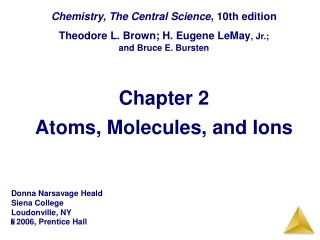# Chapter 2 Atoms, Molecules, and Ions - PowerPoint PPT PresentationDownload PresentationChapter 2 Atoms, Molecules, and Ions

Chapter 2 Atoms, Molecules, and Ions
Download Presentation## Chapter 2 Atoms, Molecules, and Ions

- - - - - - - - - - - - - - - - - - - - - - - - - - - E N D - - - - - - - - - - - - - - - - - - - - - - - - - - -
##### Presentation Transcript

1. Chapter 2Atoms, Molecules, and Ions Chemistry, The Central Science, 10th edition Theodore L. Brown; H. Eugene LeMay, Jr.; and Bruce E. Bursten Donna Narsavage Heald Siena College Loudonville, NY  2006, Prentice Hall

2. If you have equal masses of the following metals, which will have the largest number of atoms? • Pb, 207.2 g/mol • Au, 197 g/mol • Ag, 107.9 g/mol • Cu, 63.54 g/mol • Fe, 55.85 g/mol

3. If you have equal masses of the following metals, which will have the largest number of atoms? • Pb, 207.2 g/mol • Au, 197 g/mol • Ag, 107.9 g/mol • Cu, 63.54 g/mol • Fe, 55.85 g/mol

4. The mass spectrum of chlorine is shown below. How many different molar masses are possible for Cl2? • 1 • 2 • 3 • 4 • 5

5. The mass spectrum of chlorine is shown below. How many different molar masses are possible for Cl2? • 1 • 2 • 3 • 4 • 5

6. Which molar mass will be most common among Cl2 molecules? • 70 g/mol • 71 g/mol • 72 g/mol • 73 g/mol • 74 g/mol

7. Which molar mass will be most common among Cl2 molecules? • 70 g/mol • 71 g/mol • 72 g/mol • 73 g/mol • 74 g/mol

8. 1. 2. 3. 4. None of the above 5. Which of the following are have the same number of electrons (isoelectronic)?

9. 1. 2. 3. 4. None of the above 5. Which of the following are have the same number of electrons (isoelectronic)?

10. Which combination is likely to produce an ionic compound? • C and H • S and Cl • Ca and F • Br and I • Xe and F

11. Which combination is likely to produce an ionic compound? • C and H • S and Cl • Ca and F • Br and I • Xe and F

12. What are the ions present in the compound(NH4)2SO4 ? • NH3, H2, and SO2 • N3-, H+, S2-, O2- • NH42+ and SO4- • NH4+ and SO42- • (NH4+)2 and SO42-

13. What are the ions present in the compound(NH4)2SO4 ? • NH3, H2, and SO2 • N3-, H+, S2-, O2- • NH42+ and SO4- • NH4+ and SO42- • (NH4+)2 and SO42-